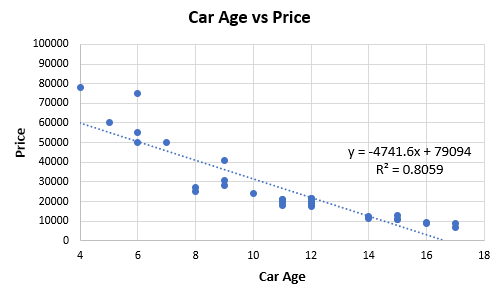# R squared Does Not Measure Predictive Capacity or Statistical AdequacyThe fact that R-squared shouldn't be used for deciding if you have an adequate model is counter-intuitive and is rarely explained clearly. This demonstration overviews how R-squared goodness-of-fit works in regression analysis and correlations, while showing why it is not a measure of statistical adequacy, so should not suggest anything about future predictive performance. The R-squared Goodness-of-Fit measure is one of the most widely available statistics accompanying the output of regression analysis in statistical software. Perhaps partially due to its widespread availability, it is also one of the most often misunderstood ones.

The R-squared Goodness-of-Fit measure is one of the most widely available statistics accompanying the output of regression analysis in statistical software. Perhaps partially due to its widespread availability, it is also one of the most often misunderstood ones.

First, a brief refresher on R-squared (R2). In a regression with a single independent variable R2 is calculated as the ratio between the variation explained by the model and the total observed variation. It is often called the coefficient of determination and can be interpreted as the proportion of variation explained by the posed predictor. In such a case, it is equivalent to the square of the correlation coefficient of the observed and fitted values of the variable. In multiple regression, it is called the coefficient of multiple determination and is often calculated using an adjustment that penalizes its value depending on the number of predictors used.

In neither of these cases, however, does R2 measure whether the right model was chosen, and consequently, it does not measure the predictive capacity of the obtained fit. This is correctly noted in multiple sources, but few make it clear that statistical adequacy is a prerequisite of correctly interpreting a coefficient of determination. Exceptions include Spanos 2019  wherein one can read “It is important to emphasize that the above statistics [R-squared and others] are meaningful only in the case where the estimated linear regression model […] is statistically adequate,” and Hagquist & Stenbeck (1998). It is even rarer to see examples of why that is the case with an exception being Ford 2015.

The present article includes a broader set of examples that elucidate the role and limitations of the coefficient of determination. To keep it manageable, only single variable regression is examined.

#### The Appeal of R-squared

First, let us examine the utility of R2 and to see why it is so easy to incorrectly interpret it as a measure of statistical adequacy and predictive accuracy when it is neither. Using a comparison with the simple linear correlation coefficient will help us understand why it behaves the way it does.

Figure 1 below is based on extracted data from 32 price offers for second-hand cars of one high-end model. The idea is to examine the relationship between car age (x-axis) and price (y-axis, in my local currency unit).

## Predictive Analytics World Berlin 2020 – Keynote Sessions Announced

Predictive Analytics World Berlin 2020: The agenda of Predictive Analytics World Berlin, 16-17 Nov, is taking shape. Curious? Take a peek at our keynote sessions 2020.

## Customer Churn Prediction: A Global Performance Study

This article details an automated machine-learned approach to predict customer churn and its results across selected communication service providers around the globe.

## 5 Big Trends in Data Analytics

Data analytics is the process by which data is deconstructed and examined for useful patterns and trends. Here we explore five trends making data analytics even more useful.

## Data Visualization in R with ggplot2: A Beginner Tutorial

Data Visualization in R with ggplot2: A Beginner Tutorial. Learn to visualize your data using R and ggplot2 in this beginner-friendly tutorial that walks you through building a chart for data analysis.